## Introduction

Imagine that you have a dataset that contains some numerical values, and you want to know the sum of a column, the maximum value of a column, or the minimum value of a column. Then, for every answer, you are required to ask different questions on Chat Bot.  Now, instead of asking different questions about maximum value, minimum value, or sum, if you can get all the answers in one place, then it can save a lot of time. This Article explains how Pivot View can be of great help. Now, for this article, you should have the know-how of Chat Bots. The Article on Chat Bot can be found here:

Pivot View

When any question is asked on Chat Bot from any dataset, there are different views generated, which are:

1. Chart View
2. Grid View
3. Pivot View

Our Focus in this article will be related to Pivot View only. The Pivot view can be found next to the Grid View: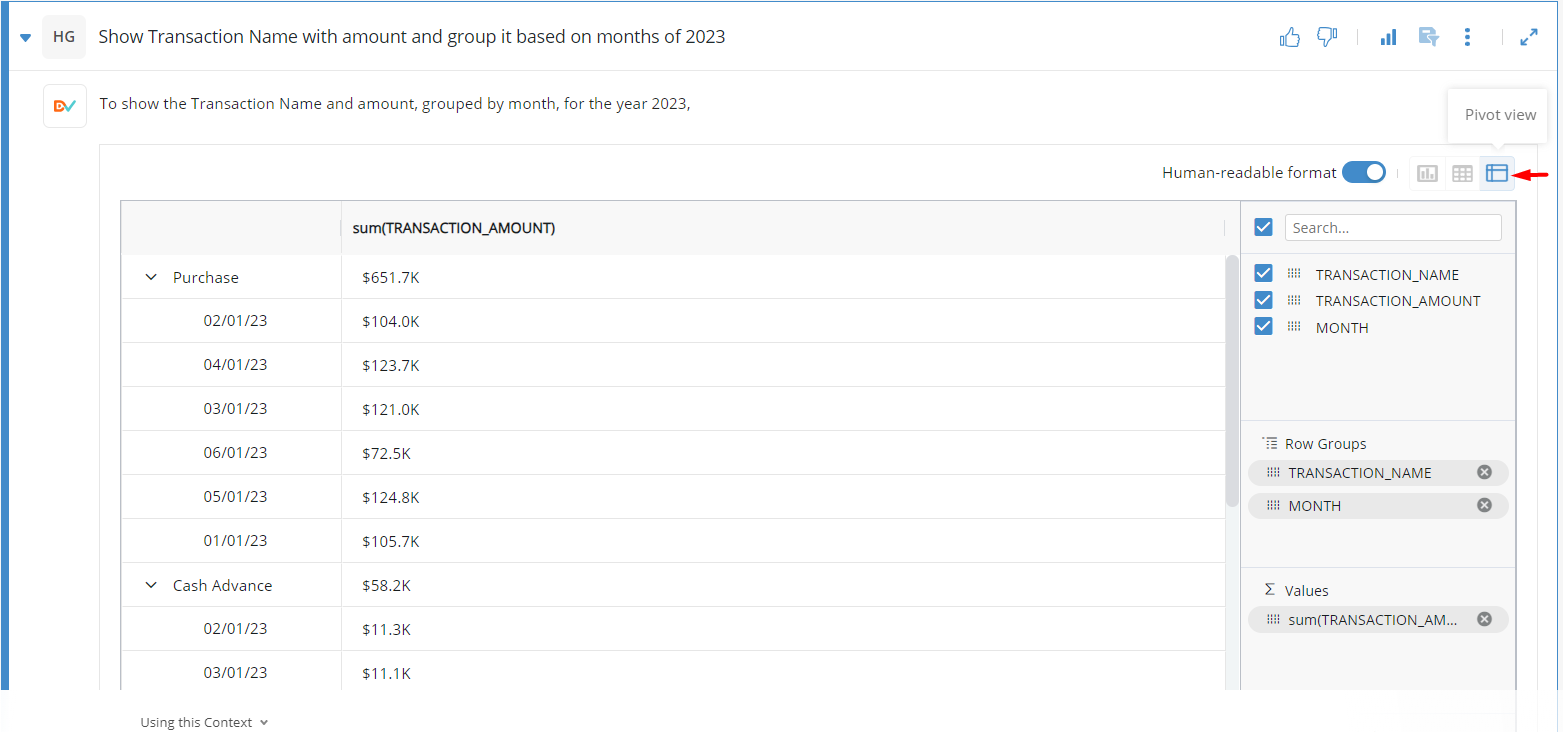Before analyzing the pivot view, let us see the grid view so we can see the data that has been brought up in the answer. The Grid View button is located to the left of the Pivot View button.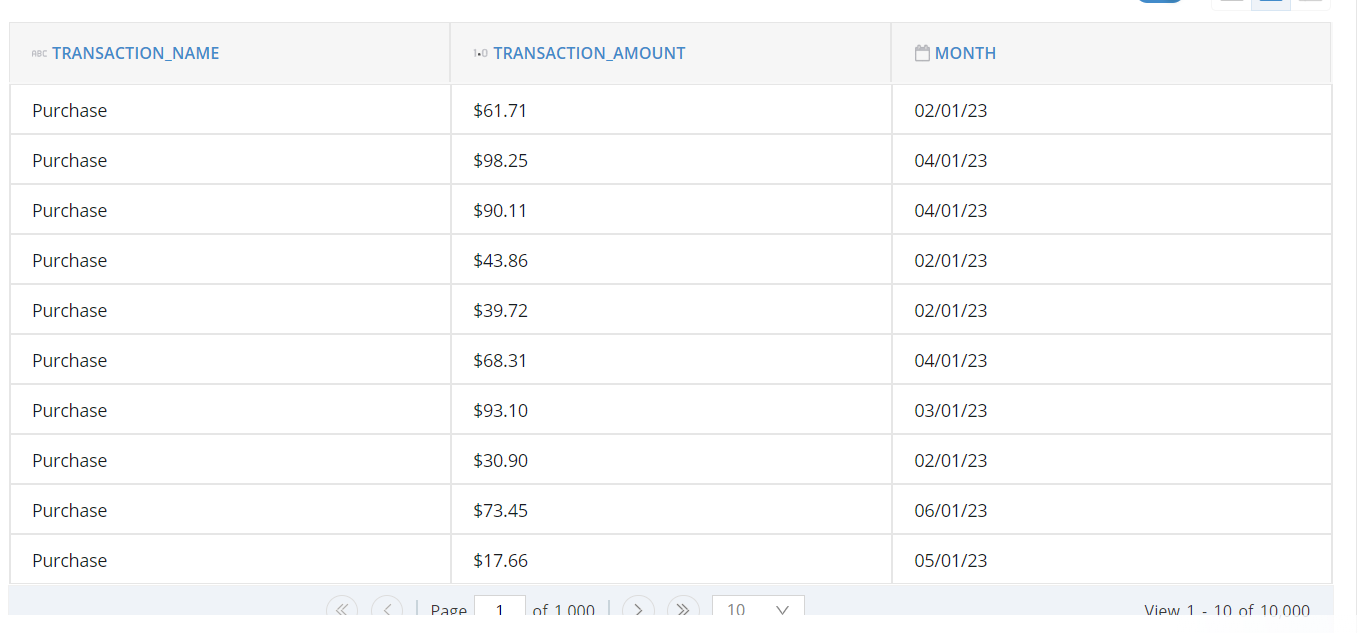In the Grid, we have Transaction Amounts and Different Transactions. Now, Imagine that for different Transaction Names, I want to know the sum of the Transaction amount or maximum value, so for that, we will be required to ask different questions. Pivot View solves this problem.

When Pivot View is opened, then on top, we have the search bar and the columns that are fetched for that question: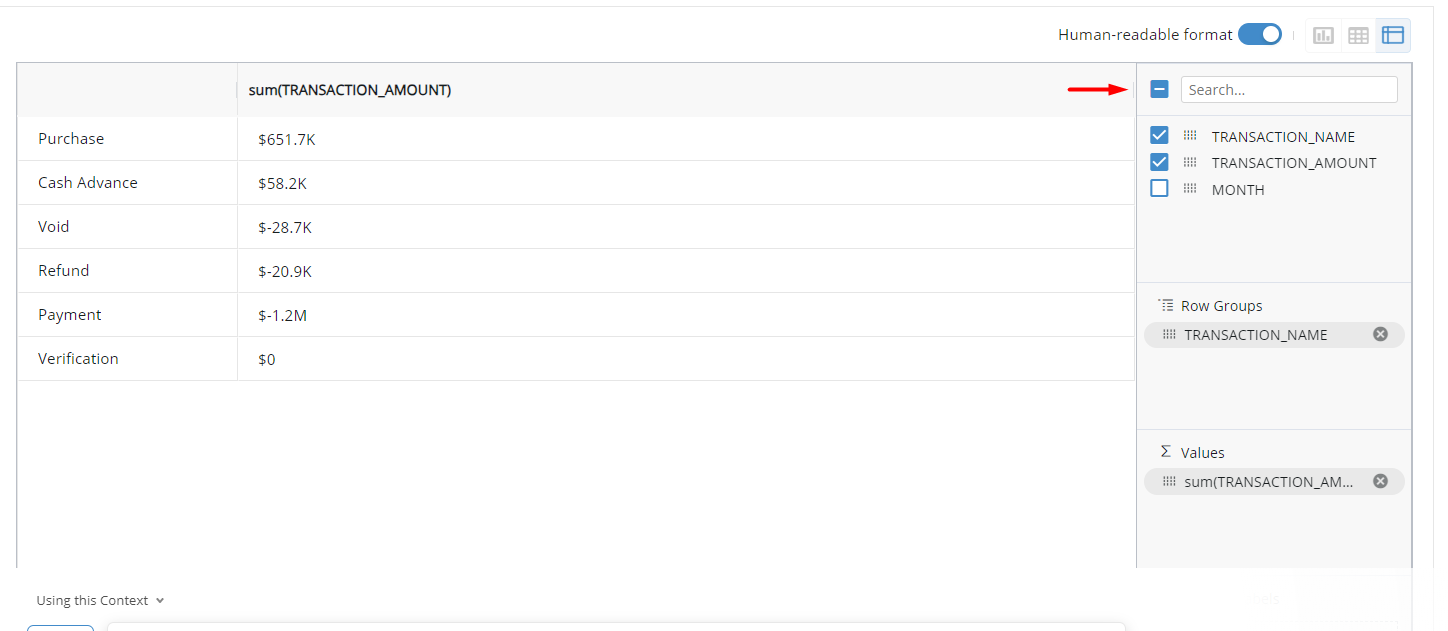Below that, we have 3 options which are:

1. Row Groups
2. Values
3. Column Groups

Row Groups

In the Row Groups, the columns that will be added will be shown as rows on the left side. The columns can be dragged from the dots present before the column name. In the Row groups, metric columns can not come under the Row Groups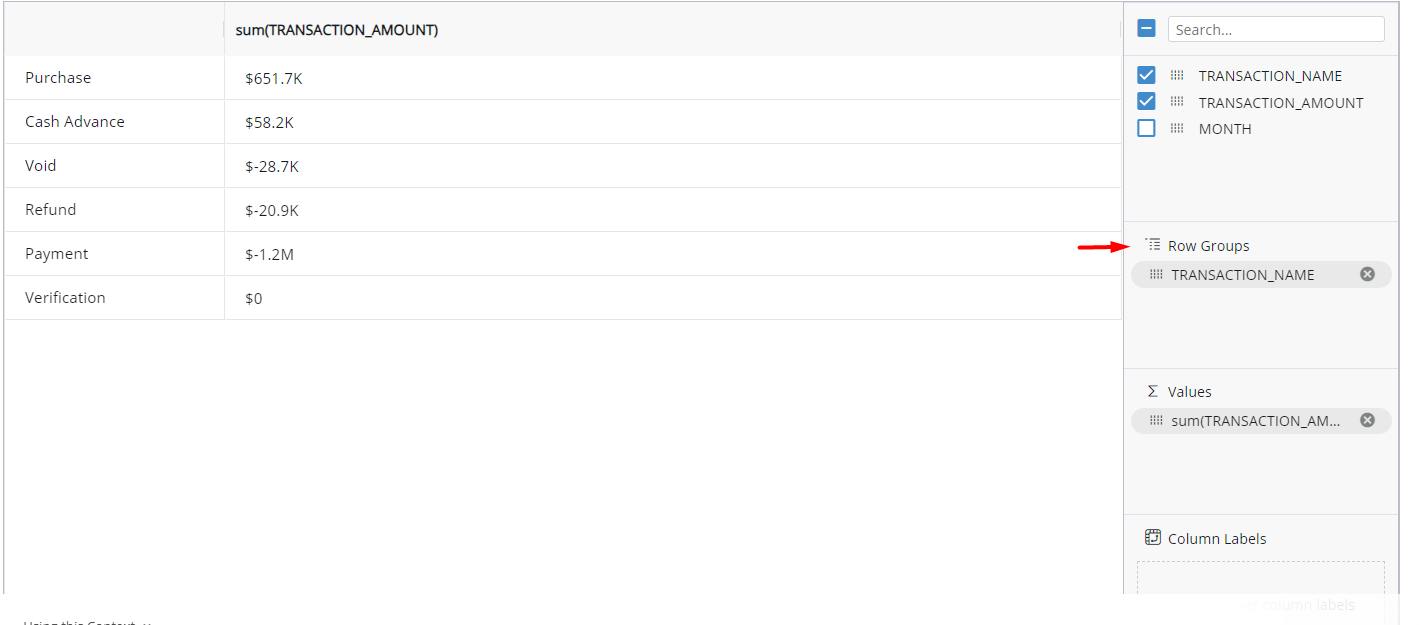Values

Under the "Values," only metric columns are allowed to be dragged. Metric columns are the columns whose data type is double, int, or decimal. Example of these types of columns is the amount, cost revenue, etc.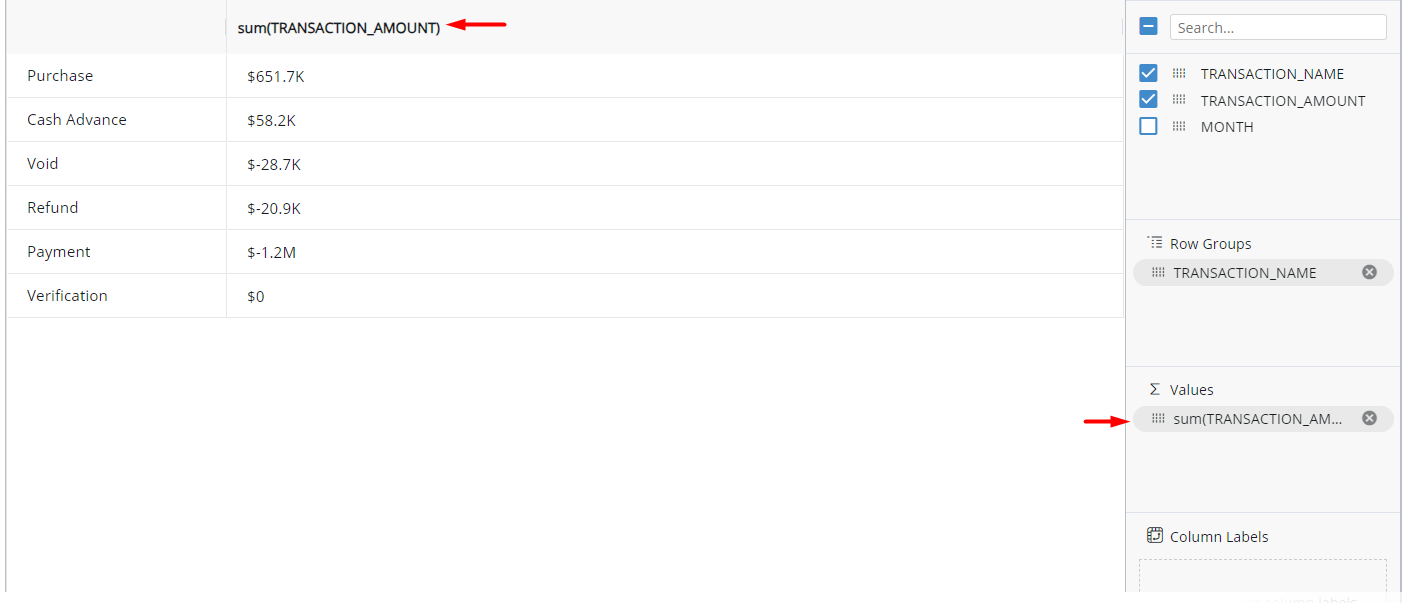If dots are clicked on the columns under "Values," then there are multiple options like sum, average, count, max, min, etc. In this way, the user can shift between them, and the grid on the left side will be changed accordingly: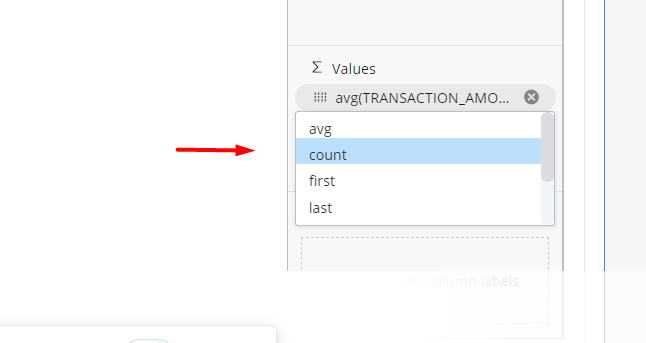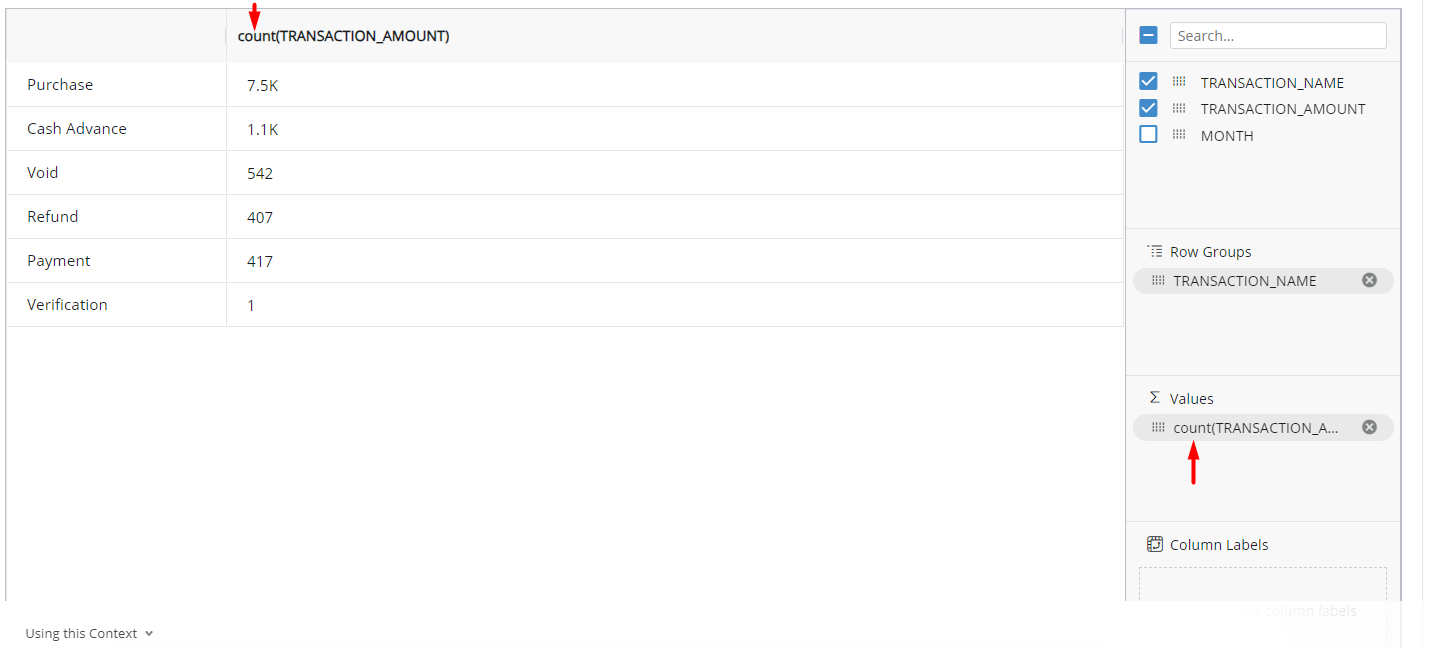Here, in one place, the user can view the average, sum, minimum value, and maximum value of a metric instead of typing 3 to 4 different questions.

Column Labels

Just like in Row groups where we added columns as rows, in the column labels, columns added here will be added on top as columns: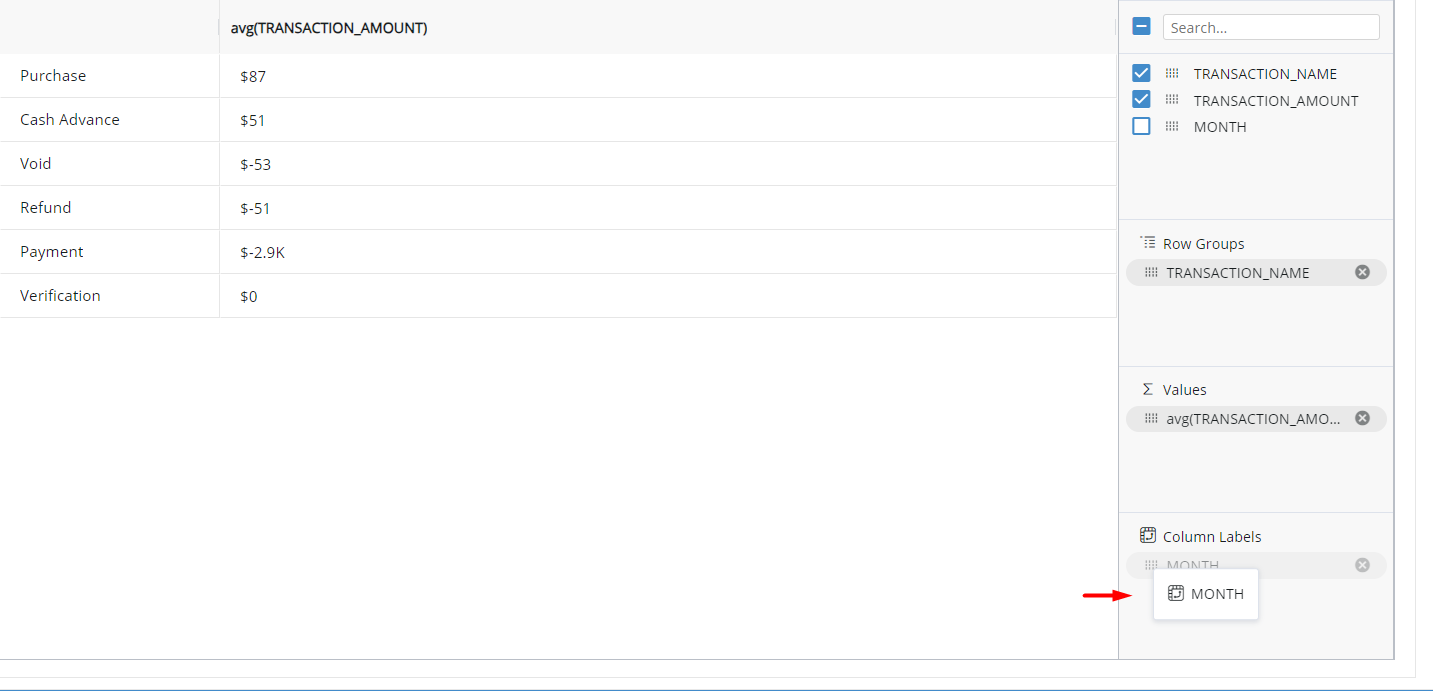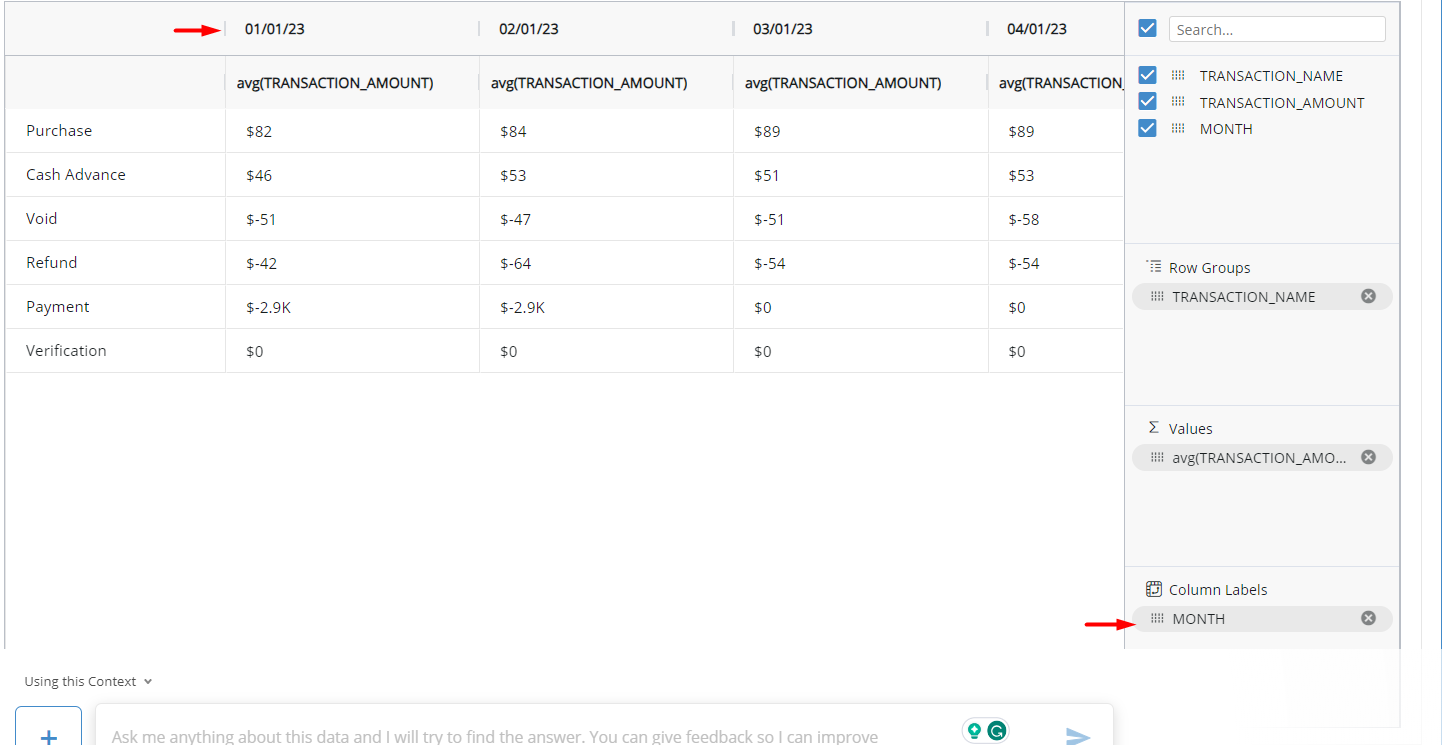Now, here for different dates and Transaction Names, the user can see the average, maximum, minimum, count, etc. of the transaction amount. That is how Pivot view saves the users from asking multiple questions. All of that information can be seen here. One thing is to be noted: just like Row Groups, metric columns are also not allowed to be dragged here.

The Pivot View is provided in both Chat Bots and on CADDI chat. For more information on Chat Bots and CADDI chat their articles can be found here: